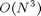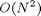# Beginner Woes

There are a few pitfalls that new users of MATLAB almost always fall into, depending on their applications. I thought it might be nice to summarize a few of them here, and, I hope, use you the readers to add to the list.

### Matrix Multiplication vs. Elementwise

From its inception, MATLAB was meant to be a MATrix LABoratory. Since initially the only datatype was a two-dimensional array, Cleve chose * for the matrix multiplication operator. To multiply two arrays elementwise, use .* instead.

A = magic(3)
B = diag([-1 1 -1])
AB = A*B
eachAB = A.*B
A =
8     1     6
3     5     7
4     9     2
B =
-1     0     0
0     1     0
0     0    -1
AB =
-8     1    -6
-3     5    -7
-4     9    -2
eachAB =
-8     0     0
0     5     0
0     0    -2


Sometimes new users type A*B and find it takes a really long time because they are expecting the elementwise output. For a square matrix of order N, and without accounting for any special characteristics (e.g., if one of the matrices is diagonal, sparse, banded, etc.), then matrix multiplication takesoperations whereas .* takes### Transpose for Complex Data

Again, going back to MATLAB's roots, the original basic datatype was not only two-dimensional, but the values were double and complex (with appropriate storage optimizations if the data were real-valued). Being the MATrix LABoratory that MATLAB is, we needed an operator for transposing a matrix and chose '. Looking at the documentation for ', we see that ' performs the complex conjugate transpose. We also see that .' performs the transpose without doing the conjugation. For real matrices, ' and .' are the same. But for complex matrices, they differ.

Ai = A+i*[1 2 3; 4 5 6; 7 8 9]
Aict = Ai'
Ait = Ai.'
Ai =
8.0000 + 1.0000i   1.0000 + 2.0000i   6.0000 + 3.0000i
3.0000 + 4.0000i   5.0000 + 5.0000i   7.0000 + 6.0000i
4.0000 + 7.0000i   9.0000 + 8.0000i   2.0000 + 9.0000i
Aict =
8.0000 - 1.0000i   3.0000 - 4.0000i   4.0000 - 7.0000i
1.0000 - 2.0000i   5.0000 - 5.0000i   9.0000 - 8.0000i
6.0000 - 3.0000i   7.0000 - 6.0000i   2.0000 - 9.0000i
Ait =
8.0000 + 1.0000i   3.0000 + 4.0000i   4.0000 + 7.0000i
1.0000 + 2.0000i   5.0000 + 5.0000i   9.0000 + 8.0000i
6.0000 + 3.0000i   7.0000 + 6.0000i   2.0000 + 9.0000i


Why does this trip people? Sometimes when I write a general algorithm, I expect it to work regardless of the data, be it real or complex. Now suppose my code is working with column vectors, but I am willing, as a convenience, to have the code handle rows as well. I just need to transform them, in this case transpose. If I am not careful here, and use ', I will conjugate the original data and I didn't mean to.

### Other Beginner Woes?

I have a few more topics in the Woes category that I will cover in a later blog. Some of them happen to new users, and some happen to even those of us with more MATLAB experience. Please post other common pitfalls you've seen new users experience here.

Published with MATLAB® 7.4

|